• matlab进行信号滤波,附带程序，几个特别经常用例子，希望大家有帮助
• 不错的matlab编写的程序语音信号进行滤波处理 在语音信号处理中非常有用的
• 心电信号处理matlab程序对学习、课程设计以及毕业设计有比较大帮助
• %实现了频率为20和200Hz单频叠加cos信号的低通滤波，使输出仅含有20Hz分量 clear; fs=1200; %采样频率 N=1200; % N/fs 秒数据 n=0:N-1; t=n/fs; %时间 fL=20; fH=200; sL=cos(2*pi*fL*t); sH=cos(2*pi*fH*t); ...
• CHANGZHOU INSTITUTE OF TECHNOLOGY 课程报告 题目 基于 MATLAB的语音信号分析及 滤波 二级学院 计算机信息工程学院 专 业 通信工程 班级 12 通信一 学生姓名 赵红梅 学号 12030735 指导教师 何松 职称 讲师 评阅...
• 一段信号的滤波程序MATLAB实现，不仅仅一种滤波
• 程序是利用matlab设计切比雪夫滤波器实现心电信号的滤波，并绘制滤波前后时域波形。
• 本材料是基于MATLAB音频信号的采样及滤波的程序及相关资料
• 巴特沃兹滤波MATLAB程序-滤波器设计.doc 学信号一个小课题一部分。 信号的采样，滤波器设计的MATLAB实现 滤掉了高频噪声 水平有些菜，希望能初学信号的同学有帮助 运行程序时候请找一个WAV文件命名 ...
• 中值滤波的特点即是首先确定一个以某个像素为中心点的邻域，一般为方形邻域，也可以为圆形、十字形等等，然后将邻域中各像素的灰度值排序，取其中间值作为中心像素灰度的新值，这里领域被称为窗口，当窗口移动时，...
中值滤波：基于排序统计理论的一种能有效抑制噪声的非线性平滑滤波信号处理技术。中值滤波的特点即是首先确定一个以某个像素为中心点的邻域，一般为方形邻域，也可以为圆形、十字形等等，然后将邻域中各像素的灰度值排序，取其中间值作为中心像素灰度的新值，这里领域被称为窗口，当窗口移动时，利用中值滤波可以对图像进行平滑处理。其算法简单，时间复杂度低，但其对点、线和尖顶多的图像不宜采用中值滤波。很容易自适应化。中值滤波对椒盐噪声抑制很好，对高斯噪声抑制一般。

clc
close all
f=[1 2 1 4 3;
1 10 2 3 4;
5 2 6 8 8;
5 5 7 0 8 ;
5 6 7 8 9];
[row, col]=size(f);
k=f;
for r=2:row-1
for c=2:col-1
m=ones(3, 3);
n=ones(3, 3);
b=k;
n(2,2)=b(r,c);    %22
n(1,1)=b(r-1,c-1);%11
n(1,2)=b(r-1,c);  %12
n(1,3)=b(r-1,c+1);%13
n(2,1)=b(r,c-1);  %21
n(3,1)=b(r+1,c-1);%31
n(3,2)=b(r+1,c);  %32
n(2,3)=b(r,c+1);  %23
n(3,3)=b(r+1,c+1); %33
out=n.*m;
mid=median(out(:));
f(r,c)=mid;
end
end

原来的f矩阵：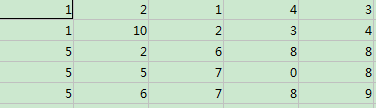中值滤波后：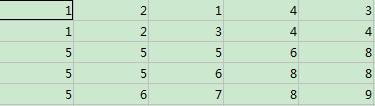%%%median函数能取一列或者一行数的中值

编的这个有点菜

问题：确定一个点，其邻域怎么定
展开全文• 常增益α-β滤波是卡尔曼滤波的简化，在信号处理及自动控制领域广泛应用。本程序仿真了常速度运动过程中位置的α-β滤波估计。
• 采用kalman滤波算法，含噪声语音信号进行降噪，能够取得较好效果。语音降噪
• MATLAB编写这篇论文中NAFSM滤波程序，强烈建议阅读此篇论文以便更好地理解论文作者思想。 需明确几点问题 在编写程序之前有几点问题要注意： 图像去噪时按照先列后行原则，这符合MATLAB在矩阵存储方式...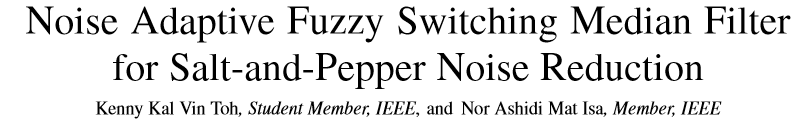用MATLAB编写这篇论文中的NAFSM滤波程序，强烈建议阅读此篇论文以便更好地理解论文作者的思想。
需明确的几点问题
在编写程序之前有几点问题要注意：

对图像去噪时按照先列后行的原则，这符合MATLAB在矩阵存储方式上为列优先存储的原则；
在去噪过程中每个像素点左上方的噪声点都是去噪并恢复了的，噪声点的灰度值一经恢复立即取代原噪声信号值，并非每个噪声点都在原图的基础上进行恢复；
求中位数要剔除过滤窗中的噪声点，这是为了避免把噪声点的灰度值选为中位数；
过滤窗中至少要有一个非噪声点，但是过滤窗最大不能超过7*7，这是为了避免把灰度值为0/255的真值点错当成噪声点导致不断扩大过滤窗的大小，所以必须对过滤窗的大小进行限制；
为了对边缘进行滤波笔者在滤波前首先进行了边缘填充，滤波结束之后再去掉填充的边缘点；

MATLAB程序
I=rgb2gray(imread('lena.jpg'));%读入图像并转化成灰度图

M=imnoise(I,'salt & pepper',0.75);%加入椒盐噪声，也可以采用input语句自己输入噪声百分比

subplot(231);imshow(I);title('original');%显示原图

subplot(232);imshow(M);title('75% salt & pepper noise');%显示噪声图

[height,width]=size(M);

s=1;%2*s+1为滤波模板的大小

salt=255;pepper=0;%salt和pepper代表噪声信号的灰度值

T1=10;T2=30;%T1和T2为模糊隶属函数中的两个门限值

P=M;%P用于进行普通中值滤波

M =padarray(M,[3,3]); %对图像边缘进行填充

N=M;%N用于剔除过滤窗中的噪声点

for j=4:width+3 %边缘点灰度值都是0不纳入此循环,循环时先列再行

for i=4:height+3

if N(i,j)==salt || N(i,j)==pepper

N(i,j)=0;%N中所有椒盐噪声点的灰度值都变成0

else

N(i,j)=1;

end

end

end

M=double(M);

for j=4:width+3 %对M进行NAFSM滤波，不考虑加上去的边缘点,循环时先列再行

for i=4:height+3

if (M(i,j)==0)||(M(i,j)==255)%找出M中的噪声点

c= N(i-s:i+s,j-s:j+s);%c的大小即filtering window的大小

a=find(c);%a是一个列向量，找出c中不为0的元素，存放过滤窗中noise-free pixel的位置

while isempty(a) && s<3 %若a为空矩阵，代表c中全部都是噪声点，此时需要扩大过滤窗，使过滤窗中含有至少一个noise-free pixel，过滤窗最大为7*7

s=s+1;

c= N(i-s:i+s,j-s:j+s);

a=find(c);

end

if isempty(a) && s==3 %&& s==3可以省略,若7*7过滤窗中无noise-free pixel，它可能是非噪声点，不过它的灰度值与噪声信号一样

E=[M(i-1,j-1),M(i,j-1),M(i+1,j-1),M(i-1,j)];%取3*3过滤窗的前四个点来计算中间值，注意，这四个点已经经过了NAFSM滤波

E=sort(E);%将E升序排列，当然，也可以直接使用库函数med=round（median(E)）求中位数

med=round((E(2)+E(3))/2);%med为中位数

else

len=length(a);%len为过滤窗中非噪声点的个数

E=zeros(1,len);%E用于存放过滤窗中所有非噪声点

c= M(i-s:i+s,j-s:j+s);

for k=1:len

E(k)=c(a(k));

end

E=sort(E);%升序排列，同样，这里也可以直接用med=round（median(E)）求中位数

if mod(len,2)==0 %length(E)为偶数时

med=round((E(len/2)+E(len/2+1))/2);%偶数个数的中位数为中间两个数的均值

else

med=E((len+1)/2);%奇数个数的中位数为中间那个数

end

end

D=abs(M(i-1:i+1,j-1:j+1)-M(i,j));

d=max(max(D)); %d为local information，3*3过滤窗中绝对亮度差的最大值

if d<10

f=0;%f为模糊隶属度，由d和预先设置的T1,T2决定，此处选择T1=10,T2=30

elseif 10<=d && d<=30

f=(d-T1)/(T2-T1);

else

f=1;

end

M(i,j)=round((1-f)*M(i,j)+f*med);%此公式计算the correction term to restore a detected“noise pixel”

end

end

end

M=M(4:height+3,4:width+3);%去掉填充的边缘

M=uint8(M);

subplot(233);imshow(M);title('NAFSM filter');%显示NAFSM滤波后的结果图

%利用MATLAB库函数进行中值滤波

P1=medfilt2(P,[3 3]);

P2=medfilt2(P,[5 5]);

P3=medfilt2(P,[7 7]);

subplot(234);imshow(P1);title('medfilter 3*3');

subplot(235);imshow(P2);title('medfilter 5*5');

subplot(236);imshow(P3);title('medfilter 7*7');

滤波结果
论文中噪声从5%加到95%，所以这里分别添加5%，25%，50%，70%，90%和95%椒盐噪声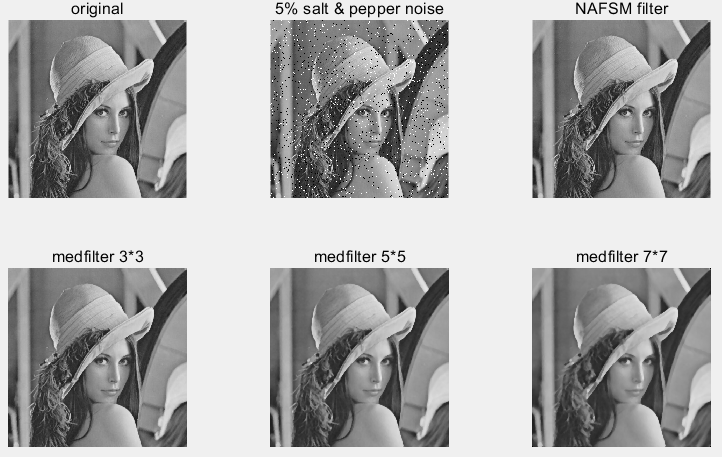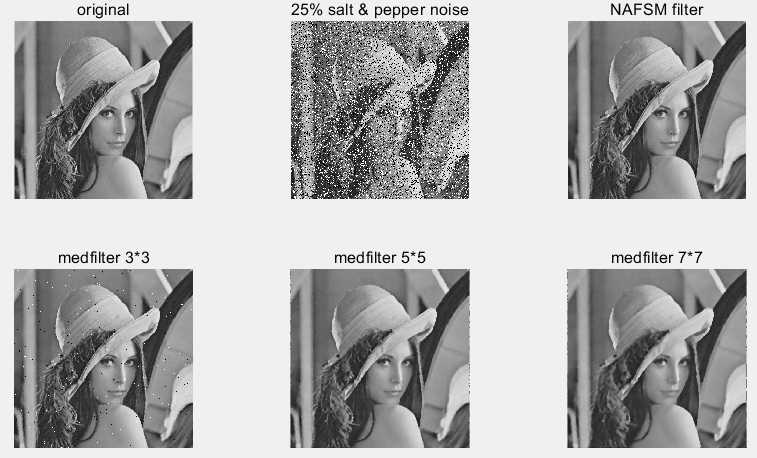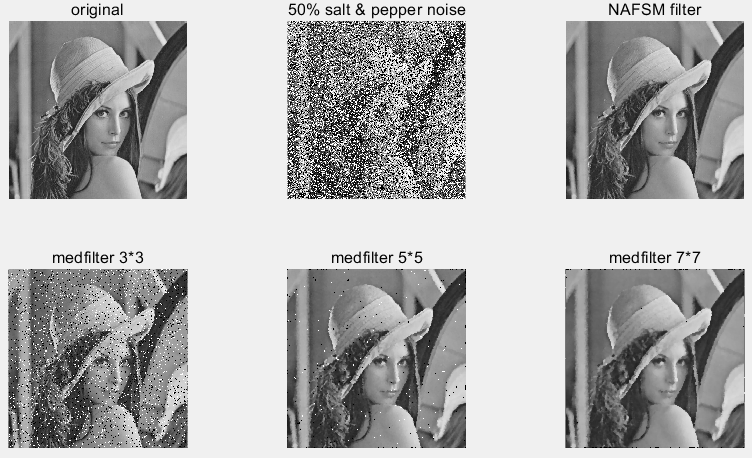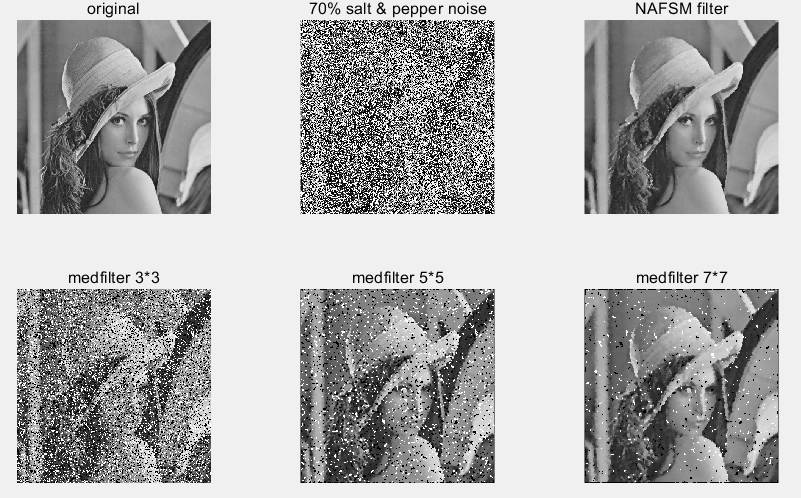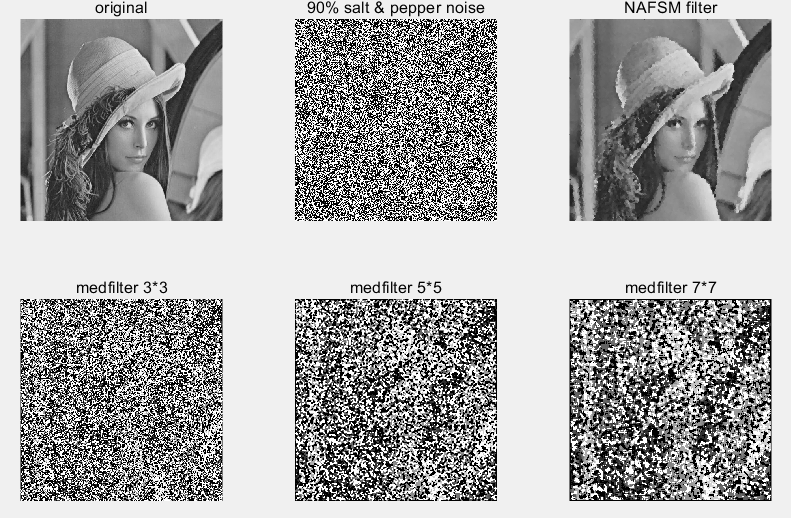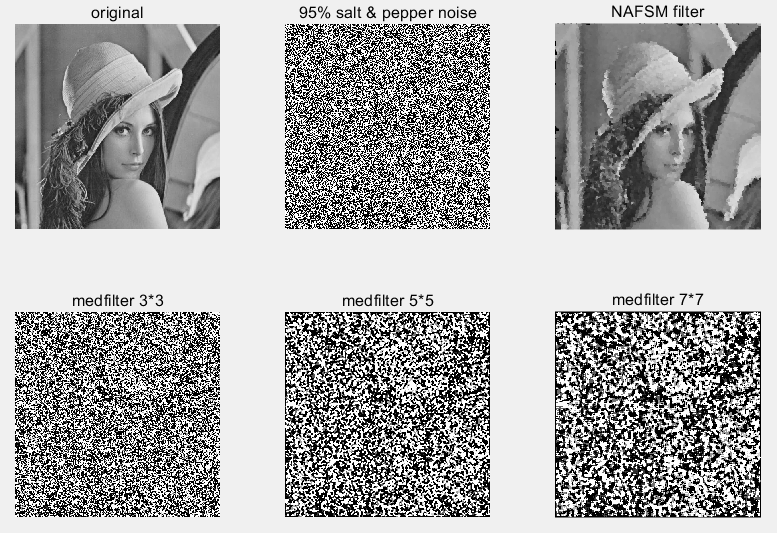总结
由滤波结果可见，NAFSM滤波比一般中值滤波效果好很多，它能有效抑制高密度椒盐噪声，同时较好地保留图像细节，轮廓和纹理，并且计算也不需要耗费太长时间。
最后，
复制有风险，粘贴需谨慎，注意躲避前方学术打假斗士XXX教授，小心翻船
Peace！


展开全文• ## 卡尔曼滤波及MATLAB程序

万次阅读 多人点赞 2018-08-24 22:27:36
转载 张文宇博客 ...KF基本思想是：采用信号、噪声、状态空间模型，利用前一时刻状态最优估计值及其误差方差估计和现时刻量测值来更新状态变量估计，求出现在时刻...
转载 张文宇的博客   https://blog.csdn.net/zhangwenyu111/article/details/17034813

卡尔曼滤波是一个很常用的滤波算法，与维纳滤波相比有很多长处。这里我们把Kalman Filter简称为KF。KF的基本思想是：采用信号、噪声、状态空间模型，利用前一时刻的状态最优估计值及其误差方差估计和现时刻的量测值来更新对状态变量的估计，求出现在时刻的最优估计值。

说白点就是对现在时刻的估计（可能是同时估计好几个变量）是取决于前一时刻估计误差和现在时刻的某个观测值。通过不断的预测和实测来修正自己的估计值，最后达到一个理想的平稳状态。

卡尔曼滤波的具体原理和推导过程，有点复杂。某些细节我也没弄明白，不过这并不影响我们写程序和使用它。如果你看了我写的这个小分享，表明你对卡尔曼滤波有一定的了解了，否则你也不会看这种玩意。如果真的不了解，那可以查找下相关资料。

卡尔曼滤波的5大公式：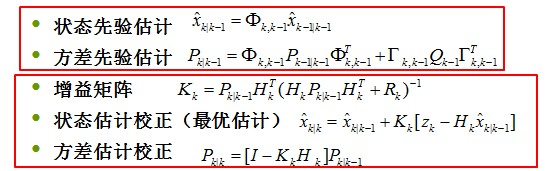这五个公式公式可要注意他们的下标了，下面我们从上至下依次说下这五个公式的意思

第一个公式就是说我们研究的变量在这一时刻与上一时刻的具体关系。那个Fai矩阵就是系数对应关系。可以看到这里就把卡尔曼滤波的使用范围限定在线性系统中了，基本的卡尔曼滤波器是不能用于非线性系统的。

第二个公式叫方差先验估计，说的这么文艺，其实就是计算估计值和实际值的方差大小，以评估现在的估计的误差是不是合适。

第三个公式叫增益矩阵，前面已经说过，卡尔曼滤波根据当前观测变量的变化趋势来估计一时刻变量的值，这个增益矩阵就是指我们定的这个变化的“度”的大小。

第四个就是求要估计的值了。

第五个是修正估计值与实际值的方差。

这5个公式是卡尔曼滤波的关键，下面就结合一个实例在说下卡尔曼滤波的程序如何写~交大的同学，如果你们选了最优估计这门课，然后看到这个，嘿嘿，便宜你们了。

应用：卡尔曼滤波在目标测量中的应用

某火箭在空中沿直线做匀加速飞行，加速度为  ，发动机推力的波动一定会引起加速度波动，雷达以间隔时间不断测量该火箭距离远点的距离  ，由于雷达的测量噪声使得火箭距离测量值   中含有噪声。现在欲通过雷达对火箭距离的测量数据求得火箭的真实距离、速度和加速度。
%%%%%%%%%%%%%%%%%%%%%%%%%%%%%%%%%%%%%%%%%%%%%%%%%%%%%%%%%
%     设置初始化信息
%  N：设置卡尔曼滤波器追踪点数
%  r：设置估计变量个数，这里r=3
%  s：被追踪的火箭的距离，初始值为1000m
%  v：火箭的速度，初始值为50m/s
%  a：火箭的加速度，初始值为20m/s2，此时加速度默认为不变
%  T: 雷达的扫描间隔，此时设为1秒
%  wt: 系统噪声，方差为20
%  vt: 量测噪声，方差为16
%%%%%%%%%%%%%%%%%%%%%%%%%%%%%%%%%%%%%%%%%%%%%%%%%%%%%%%%%
clear all;
close all;
N = 100;
r = 3;
t = 1:1:N;
T = 1;
s = zeros(1,N);
v = zeros(1,N);
a = zeros(1,N);
a(t) = 20;
s0 = 1000;
v0 = 50;
for n = 1:N
v(n) = v0 + a(n)*n;
s(n) = 1000+v0*n+0.5*a(n)*n*n;
end
wt = randn(1,N);
wt = sqrt(4)*wt./std(wt);
s = s + wt;
v = v + wt;
a(t) = a(t) + wt(t);
%%%%%%%%%%%%%%%%%%%%%%%%%%%%%%%%%%%%%%%%%%%%%%%%%%%%%%%%%
% 卡尔曼滤波部分，继承之前初始化变量
% A：转移矩阵
% H：量测矩阵
% Qk：系统噪声矩阵
% Rk：量测噪声矩阵
% P0：均方误差矩阵初始值
% Y：火箭的状态矩阵，由k_s，k_v,k_a组成
% Y0：状态矩阵的初始值
%%%%%%%%%%%%%%%%%%%%%%%%%%%%%%%%%%%%%%%%%%%%%%%%%%%%%%%%%
Y0 = [900 0 0]';
Y = [Y0 zeros(r,N-1)];
A = [1 1 0;0 1 1;0 0 1];
H = [1 0 0];
Qk = [0 0 0;0 0 0;0 0 20];
Rk = 16;
P0 = [30 0 0;0 20 0;0 0 10];
P1 = P0;
P2 = zeros(r,r);
for k = 1:N
Y(:,k) = A*Y(:,k);
P2 = A*P1*A'+Qk;
Kk = P2*H'*inv(H*P2*H'+Rk);
Y(:,k+1) = Y(:,k)+Kk*(s(:,k)-H*Y(:,k));
P1 = (eye(r,r)-Kk*H)*P2;
end
k_s = Y(1,:);
k_v = Y(2,:);
k_a = Y(3,:);
subplot(3,1,1);
plot(t,s(t),'-',t,k_s(t),'o');
title('距离');
legend('实际值','估计值');
xlabel('t');
ylabel('s');
subplot(3,1,2);
plot(t,v(t),t,k_v(t),'+');
title('速度');
legend('实际值','估计值');
xlabel('t');
ylabel('v');
subplot(3,1,3);
plot(t,a(t),t,k_a(t),'-x');
title('加速度');
legend('实际值','估计值');
xlabel('t');
ylabel('a');
axis([0,N,0,30]);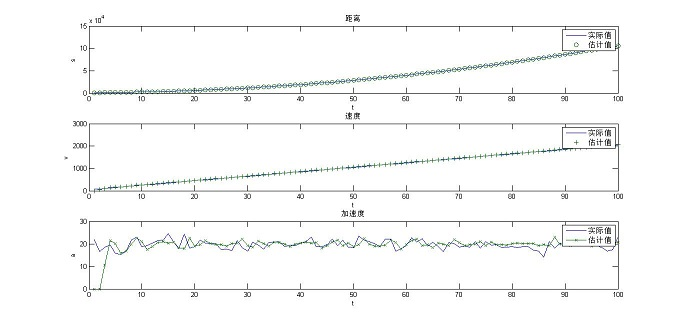展开全文• ## 卡尔曼滤波及其MATLAB程序

万次阅读 多人点赞 2013-11-30 01:14:23
今天写了个卡尔曼滤波的程序，希望有需要的同学有点帮助。 卡尔曼滤波是一个很常用的滤波算法，与维纳滤波相比有很多长处。这里我们把Kalman Filter简称为KF。KF的基本思想是：采用信号、噪声、状态空间模型，...

	今天写了个卡尔曼滤波的小程序，希望对有需要的同学有点帮助。

卡尔曼滤波是一个很常用的滤波算法，与维纳滤波相比有很多长处。这里我们把Kalman Filter简称为KF。KF的基本思想是：采用信号、噪声、状态空间模型，利用前一时刻的状态最优估计值及其误差方差估计和现时刻的量测值来更新对状态变量的估计，求出现在时刻的最优估计值。
说白点就是对现在时刻的估计（可能是同时估计好几个变量）是取决于前一时刻估计误差和现在时刻的某个观测值。通过不断的预测和实测来修正自己的估计值，最后达到一个理想的平稳状态。
卡尔曼滤波的具体原理和推导过程，有点复杂。某些细节我也没弄明白，不过这并不影响我们写程序和使用它。如果你看了我写的这个小分享，表明你对卡尔曼滤波有一定的了解了，否则你也不会看这种玩意。如果真的不了解，那可以查找下相关资料。
卡尔曼滤波的5大公式：这五个公式公式可要注意他们的下标了，下面我们从上至下依次说下这五个公式的意思
第一个公式就是说我们研究的变量在这一时刻与上一时刻的具体关系。那个Fai矩阵就是系数对应关系。可以看到这里就把卡尔曼滤波的使用范围限定在线性系统中了，基本的卡尔曼滤波器是不能用于非线性系统的。
第二个公式叫方差先验估计，说的这么文艺，其实就是计算估计值和实际值的方差大小，以评估现在的估计的误差是不是合适。
第三个公式叫增益矩阵，前面已经说过，卡尔曼滤波根据当前观测变量的变化趋势来估计一时刻变量的值，这个增益矩阵就是指我们定的这个变化的“度”的大小。
第四个就是求要估计的值了。
第五个是修正估计值与实际值的方差。
这5个公式是卡尔曼滤波的关键，下面就结合一个实例在说下卡尔曼滤波的程序如何写~交大的同学，如果你们选了最优估计这门课，然后看到这个，嘿嘿，便宜你们了。

应用：卡尔曼滤波在目标测量中的应用
某火箭在空中沿直线做匀加速飞行，加速度为  ，发动机推力的波动一定会引起加速度波动，雷达以间隔时间不断测量该火箭距离远点的距离  ，由于雷达的测量噪声使得火箭距离测量值   中含有噪声。现在欲通过雷达对火箭距离的测量数据求得火箭的真实距离、速度和加速度。

%%%%%%%%%%%%%%%%%%%%%%%%%%%%%%%%%%%%%%%%%%%%%%%%%%%%%%%%%
%     设置初始化信息
%  N：设置卡尔曼滤波器追踪点数
%  r：设置估计变量个数，这里r=3
%  s：被追踪的火箭的距离，初始值为1000m
%  v：火箭的速度，初始值为50m/s
%  a：火箭的加速度，初始值为20m/s2，此时加速度默认为不变
%  T: 雷达的扫描间隔，此时设为1秒
%  wt: 系统噪声，方差为20
%  vt: 量测噪声，方差为16
%%%%%%%%%%%%%%%%%%%%%%%%%%%%%%%%%%%%%%%%%%%%%%%%%%%%%%%%%
clear all;
close all;
N = 100;
r = 3;
t = 1:1:N;
T = 1;
s = zeros(1,N);
v = zeros(1,N);
a = zeros(1,N);
a(t) = 20;
s0 = 1000;
v0 = 50;
for n = 1:N
v(n) = v0 + a(n)*n;
s(n) = 1000+v0*n+0.5*a(n)*n*n;
end
wt = randn(1,N);
wt = sqrt(4)*wt./std(wt);
s = s + wt;
v = v + wt;
a(t) = a(t) + wt(t);
%%%%%%%%%%%%%%%%%%%%%%%%%%%%%%%%%%%%%%%%%%%%%%%%%%%%%%%%%
% 卡尔曼滤波部分，继承之前初始化变量
% A：转移矩阵
% H：量测矩阵
% Qk：系统噪声矩阵
% Rk：量测噪声矩阵
% P0：均方误差矩阵初始值
% Y：火箭的状态矩阵，由k_s，k_v,k_a组成
% Y0：状态矩阵的初始值
%%%%%%%%%%%%%%%%%%%%%%%%%%%%%%%%%%%%%%%%%%%%%%%%%%%%%%%%%
Y0 = [900 0 0]';
Y = [Y0 zeros(r,N-1)];
A = [1 1 0;0 1 1;0 0 1];
H = [1 0 0];
Qk = [0 0 0;0 0 0;0 0 20];
Rk = 16;
P0 = [30 0 0;0 20 0;0 0 10];
P1 = P0;
P2 = zeros(r,r);
for k = 1:N
Y(:,k) = A*Y(:,k);
P2 = A*P1*A'+Qk;
Kk = P2*H'*inv(H*P2*H'+Rk);
Y(:,k+1) = Y(:,k)+Kk*(s(:,k)-H*Y(:,k));
P1 = (eye(r,r)-Kk*H)*P2;
end
k_s = Y(1,:);
k_v = Y(2,:);
k_a = Y(3,:);
subplot(3,1,1);
plot(t,s(t),'-',t,k_s(t),'o');
title('距离');
legend('实际值','估计值');
xlabel('t');
ylabel('s');
subplot(3,1,2);
plot(t,v(t),t,k_v(t),'+');
title('速度');
legend('实际值','估计值');
xlabel('t');
ylabel('v');
subplot(3,1,3);
plot(t,a(t),t,k_a(t),'-x');
title('加速度');
legend('实际值','估计值');
xlabel('t');
ylabel('a');
axis([0,N,0,30]);
具体细节不讲了。运行后，就得到我们想要的结果了~一个小总结，希望对你有帮助。


展开全文Kalman
• 使用Matlab设计相应IIR数字滤波器（低通、带通或带阻等滤波器中一种）并得到滤波器H(z)系数，然后根据这些系数，编写DSP程序（C语言或汇编）已采集信号进行处理，最后在CCS软件中得到处理后音频信号的频谱图...
• MATLAB 语音信号处理之显示频谱、滤波显示原始语音信号的特征频带（谱峰位置）设计低通滤波器语音信号进行处理 显示原始语音信号的特征频带（谱峰位置） 利用Windows自带录音机录制了一个16s语音文件，编写...语音识别
• 小波去噪： load leleccum; index = 1:1024; x = leleccum(index); %产生噪声信号 init = 2055615866; randn(‘seed’,init);...%对信号进行消噪 xd1 = wdencmp(‘gbl’,nx,‘db4’,1,thr,sorh,keepapp); xd2 = wden
• 课程设计任务书 题目 基于 MATLAB 的信号消噪处理程序设计 ...造的信号加入干扰噪声加入噪声的信号进行频谱分析利用 MATLAB 含噪的信号进行滤波画出滤波信号的时域波形和频谱并对滤波 前后的信号进行对比分析信
• 中值滤波：基于排序理论一种能有效抑制噪声非线性信号处理技术。 方法：取含有基数（偶数会有半像素差）个模板数据滑动模板，模板中数据从小到大排序，取排在中间位置上数据作为最终处理结果。 ...
• 卡尔曼滤波的介绍及一些相关程序对信号处理比较有用，MATLAB语言的编程。KALMAN
• 这是用MATLAB编写的一个用于心电信号滤波去噪，和提取特征值的程序，这个是自己弄的程序，希望大家有用
• matlab信号与系统中应用-matlab信号与系统中应用.rar 此附件是关于matlab信号与系统中应用 并有各例题幻灯片 希望大家有帮助 具体实例如下: 第 6章 matlab信号与系统中应用 例6.1连续...
• 程序目的：利用维纳滤波对模糊图像进行修复，其需要顾及图像信噪比（SNR）和噪信比(NSR)，信号的功率谱使用图像方差近似估计，噪声分布已知，可以很好恢复运动模糊图像。采用deconvwnr函数实现。 输入：模糊...
• 摘要非结构化场景、重要特征提取、传感器不精确性以及无法使用GPS信号是水下环境中遇到一些挑战。在这种不利环境下，SLAM试图在未知水下环境中有效地定位机器人，同时生成环境代表性模型。介绍目前，水下...
• 关于用MATLAB设计对信号进行频谱分析和滤波处理的程序
• 文件包含了matlab程序及从MIT数据库下载文件，心电信号进行了低通、带陷、线性滤波处理，去除了干扰信号及进行了基线漂移纠正。
• 有小伙伴看了我那篇滤波的文章后，问我如何进行信号去噪，今天来给大家分享一下信号去噪入门知识。实际上，信号去噪的入门基本上就是熟练的使用各种滤波器。通常，我们通过采集卡来采集所需要的振动、声音、电流或者...
• 通过matlab对数字信号进行滤波器设计后，我们要将设计好参数放到嵌入式系统中进行滤波。 IIR滤波器 FIR滤波器 http://www.laomaotao.net/?I3254 #include <stdio.h> #include <malloc.h> //...
• 自己整理数字信号处理相关matlab程序，并重要语句进行了解释、注解。设计卷积运算，滤波，滤波器设计，以及极零图分析等有关数字信号处理的matlab文件。...

# matlab对信号滤波的程序matlab 订阅